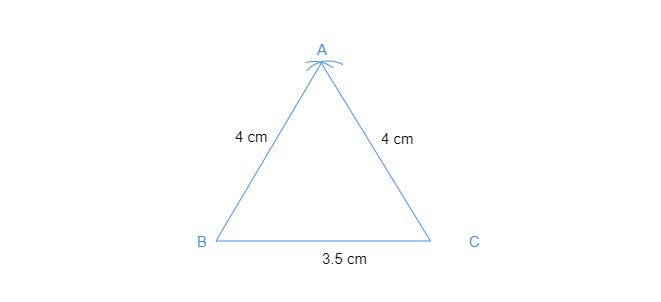# Draw $Ă˘Ë†â€ PQR$ with $PQ=4\ cm$, $QR=3.5\ cm$ and $PR=4\ cm$. What type of triangle is this?

Steps of construction -• Draw a line segment QR of length 3.5 cm.
• From Q, we need a point P is at a distance of 4 cm. So, with Q as the center, draw an arc of radius 4 cm (Now P will be somewhere on this arc. Our job is to find where exactly P is).
From R, we know that point P is at a distance of 4 cm. So, with R as the center, draw an arc of radius 4cm (Now P will be somewhere on this arc, we have to fix it).
P has to be on both the arcs drawn. So, it is the point of intersection of arcs. Mark the point of intersection of arcs as P
Join PQ and PR.
Thus, PQR is the required triangle.
ΔPQR is an isosceles triangle as two of its sides are equal, i.e., PQ = PR = 4 cm